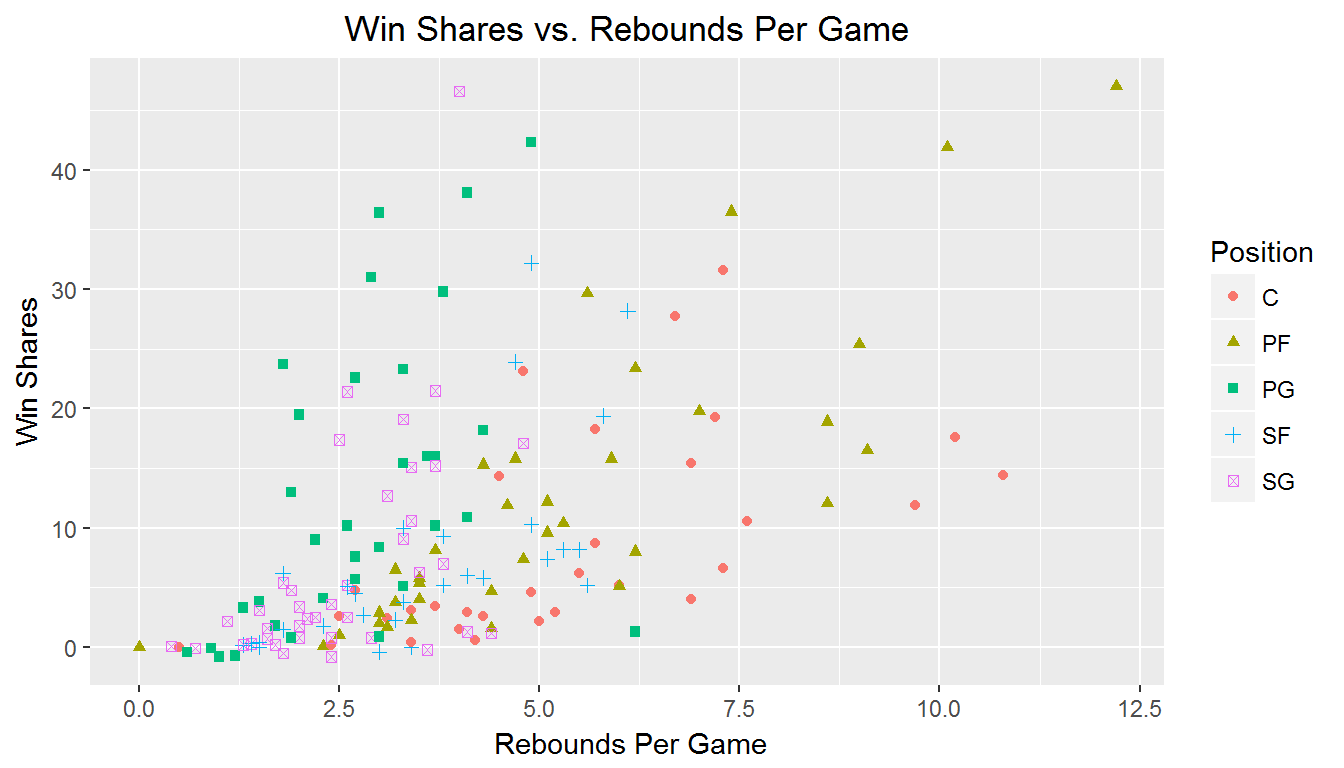## Outline

• Layers
• ggplot() vs. qplot()

## Data

We will be using the NBA draft data set.

nba <- read.csv("NBA Draft Class.csv")

## Layering

This data has the same context - a common time and common place

• Want to aggregate information from different sources onto a common plot
• With ggplot2 we will superimpose data onto this grid in layers

## Layers

To give you an idea…

library(ggplot2)
p <- ggplot() # Empty canvas
pp <- p + geom_point(data = nba,
aes(x = Points.Per.Game, y = Win.Share, colour = Year),
show.legend = T)
p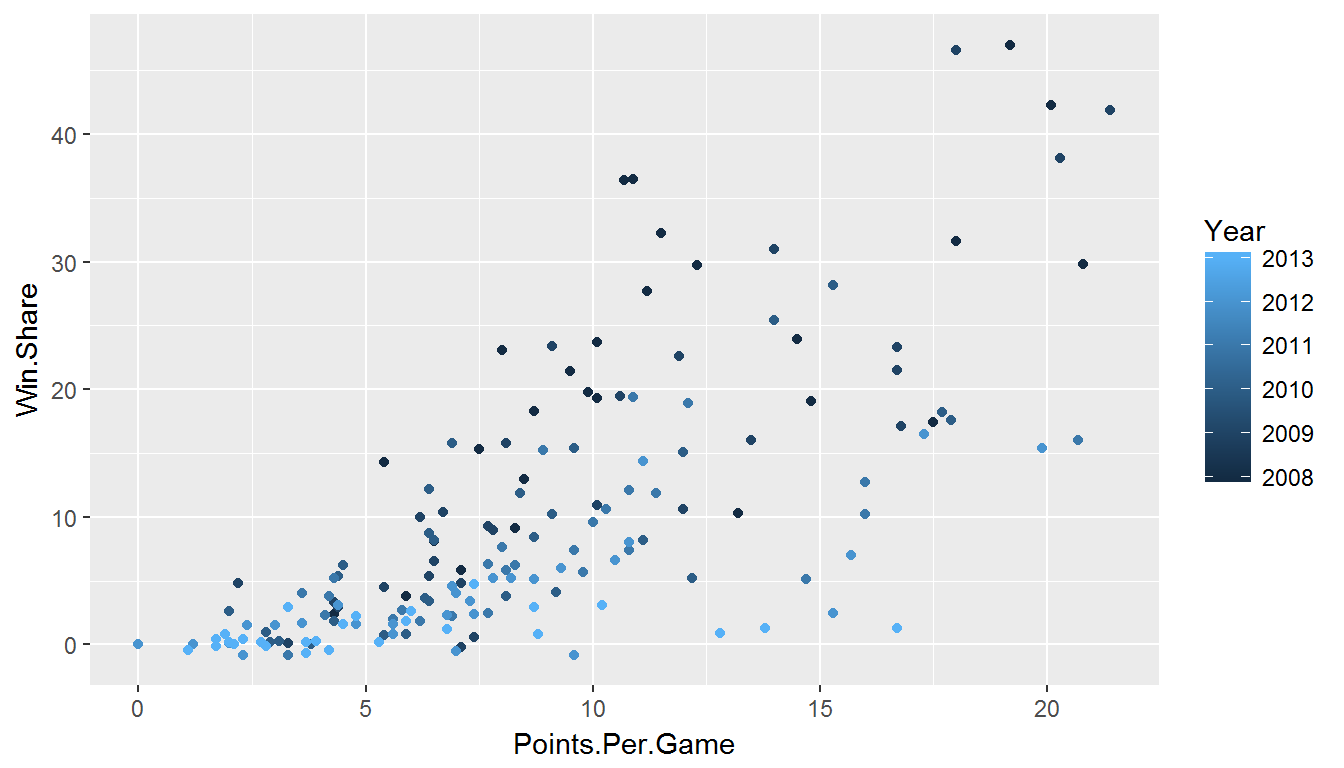Now we change the color scale of the points

p <- p + scale_colour_gradient(high = c("blue","green"))
p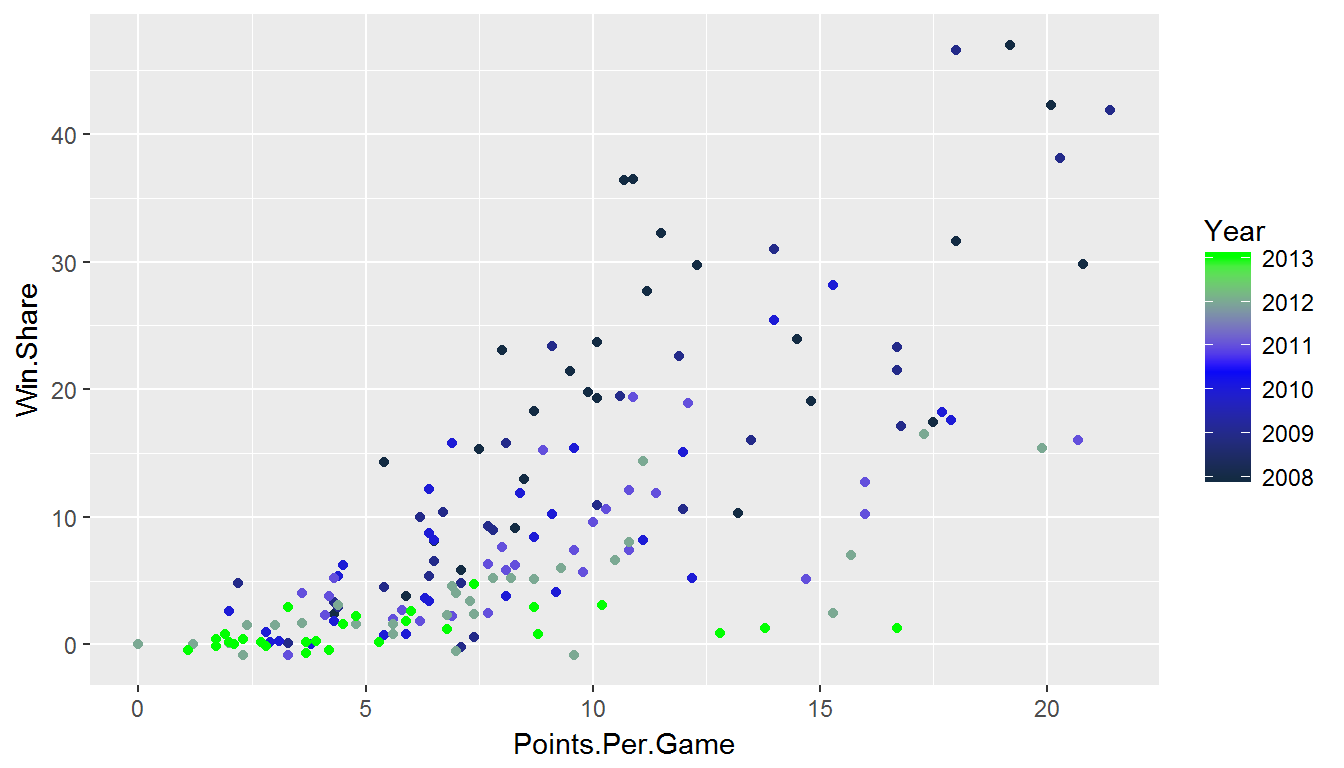p <- p + ggtitle("Win Shares vs Points Per Game")
p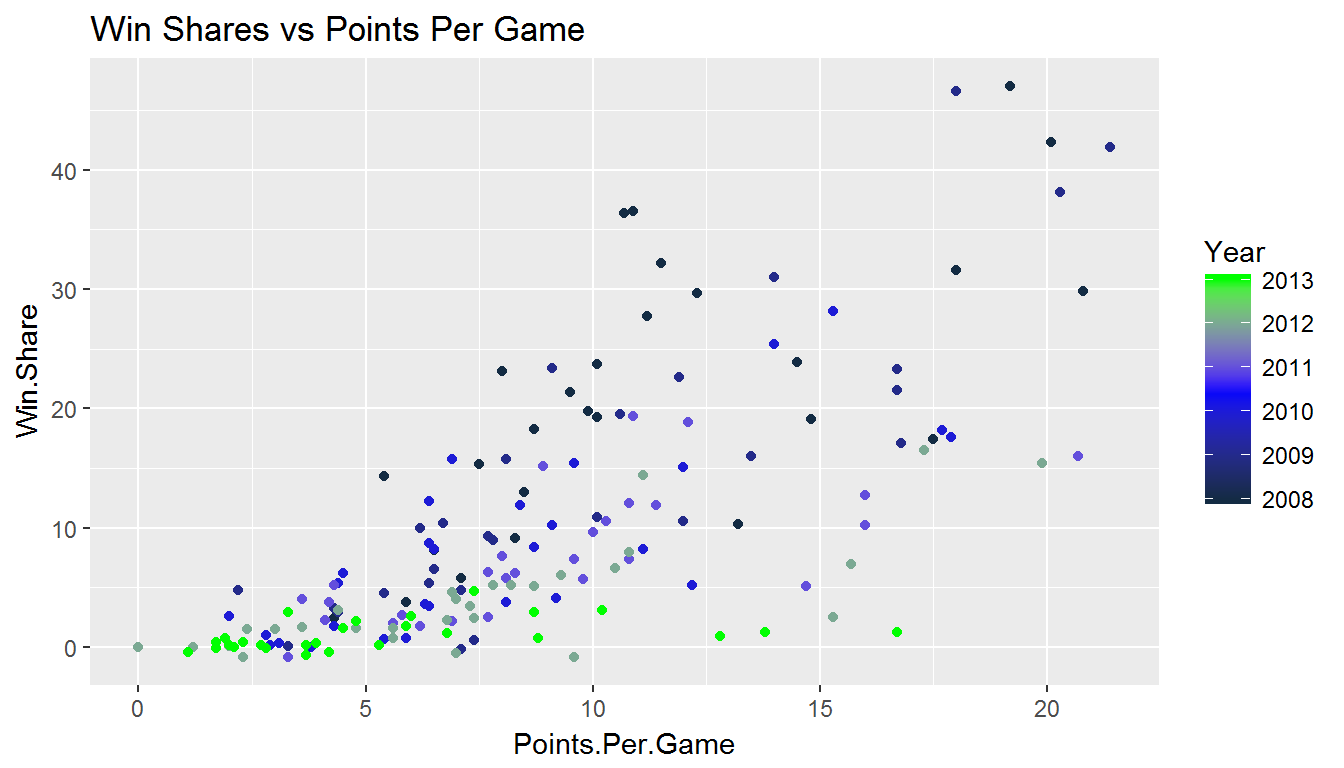p <- p + labs(x = "Points Per Game", y = " Win Shares")
p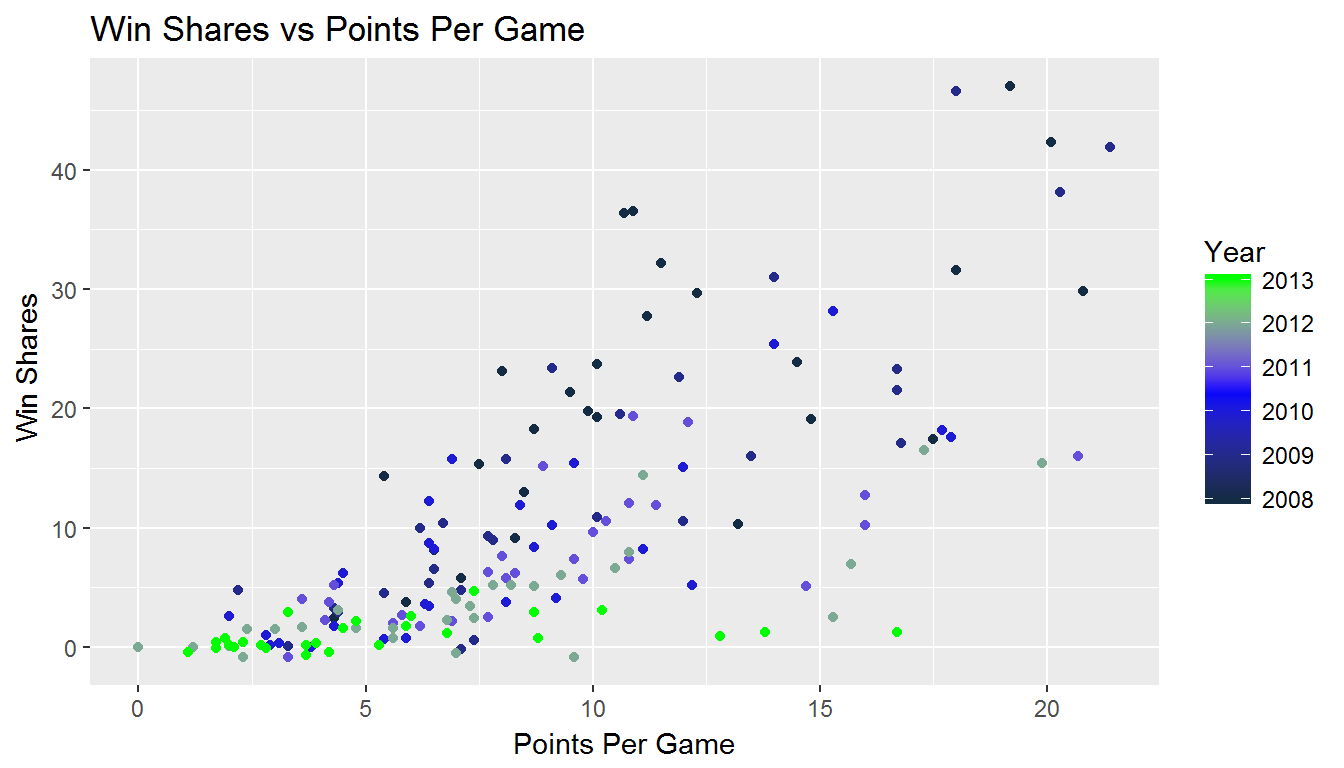Now we edit some ascthetics

p <- p + theme(plot.title =
element_text(hjust = .5, face = "bold", colour = "blue", size = 25))
p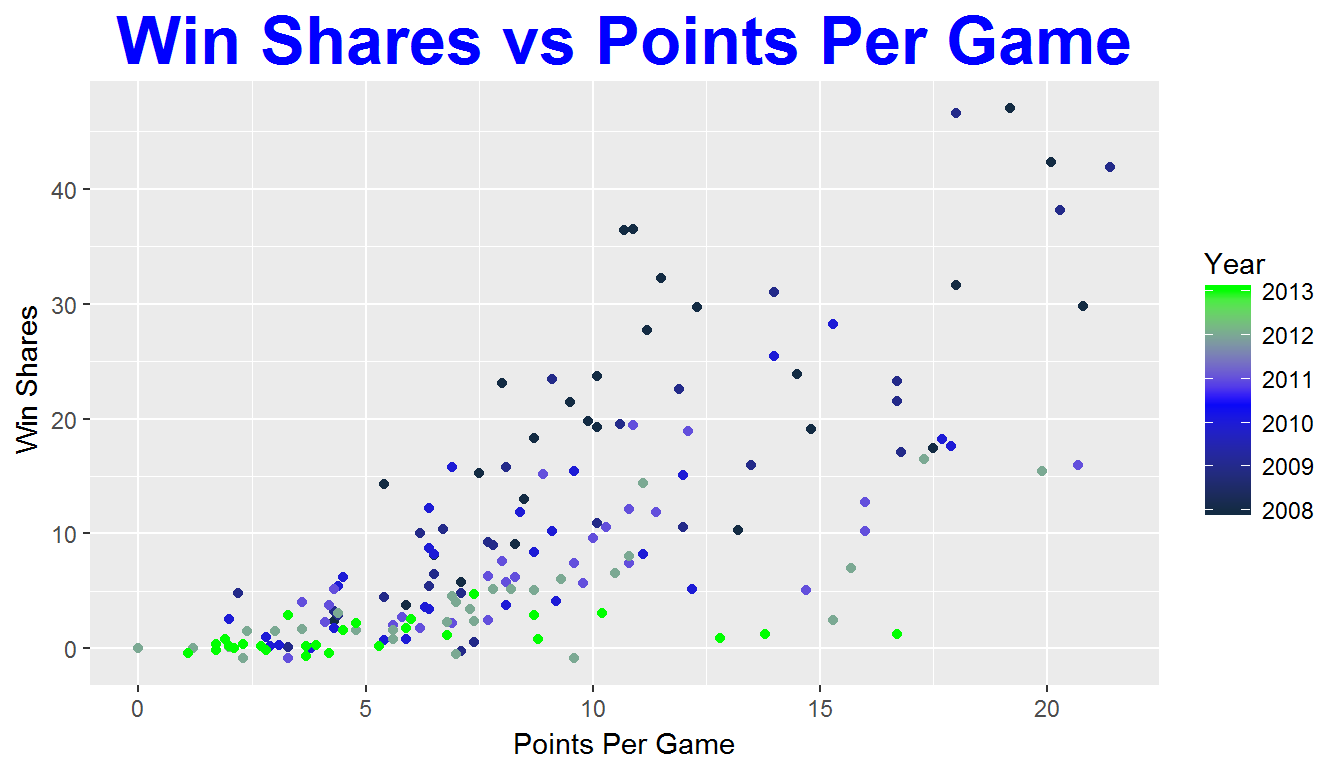## More Layering

• Most maps (and many plots) have multiple layers of data. The layers may be from the same or different datasets.
• ggplot2 builds around this same idea. Very easy to add additional layers to the plot. To do this we need to understand a little more about the underlying theory…

## What is a Plot?

• A default dataset
• A coordinate system
• layers of geometric objects (geoms)
• A set of aesthetic mappings (taking information from the data and converting into an attribute of the plot)
• A scale for each aesthetic
• A facetting specification (multiple plots based on subsetting the data)

## qplot() vs. ggplot()

qplot() stands for "quickplot":

• Automatically chooses default settings to make life easier
• Less control over plot construction

ggplot() stands for "grammar of graphics plot"

• Contructs the plot using components listed in previous slides
• Very flexible

## qplot() vs. ggplot()

Different ways to construct the same plot:

qplot(Points.Per.Game, Win.Share, colour = Year, data = nba,
main = "Win Shares vs. Points Per Game")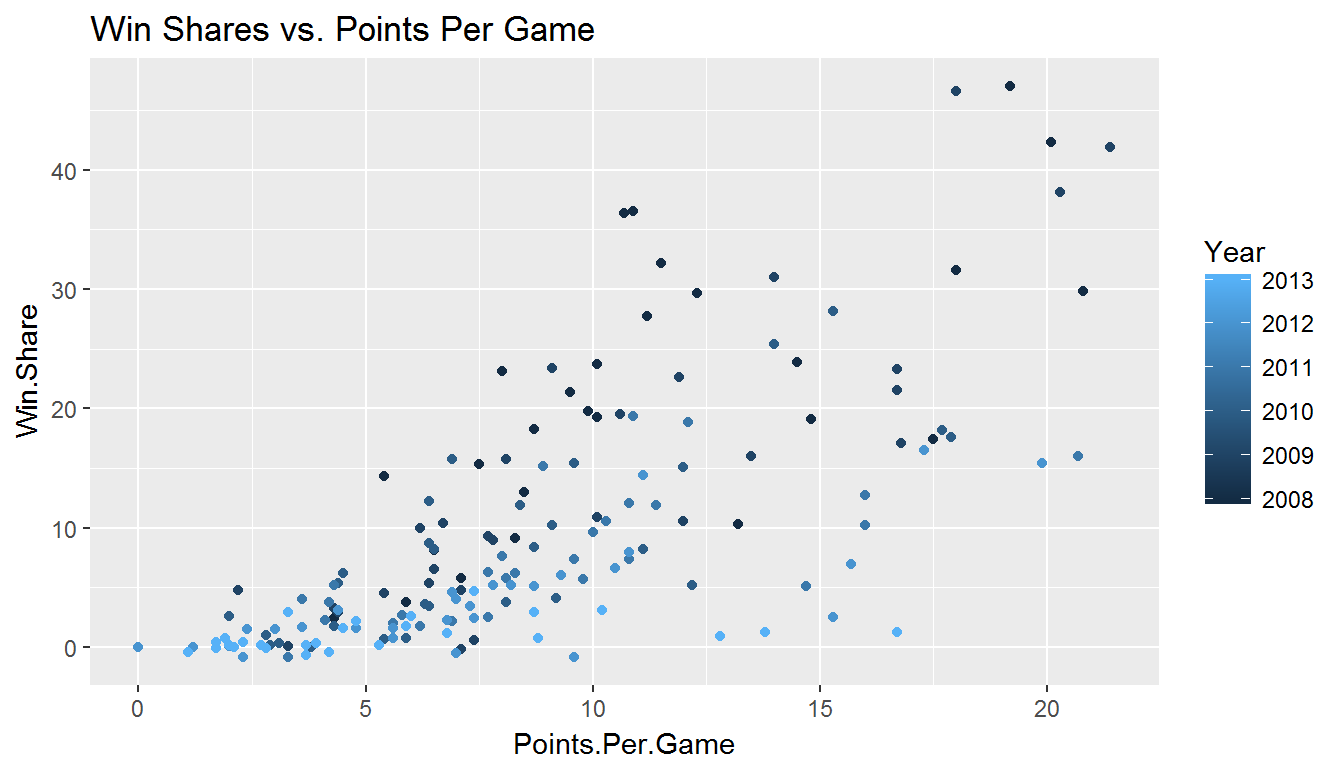or:

ggplot() +
geom_point(data = nba,
aes(x = Points.Per.Game,
y = Win.Share, colour = Year),
show.legend = T) +
ggtitle("Win Shares vs. Points Per Game")even this works:

ggplot(data = nba,
aes(x = Points.Per.Game, y = Win.Share, colour = Year)) +
geom_point()+
ggtitle("Win Shares vs. Points Per Game")## What is a Layer?

A layer added ggplot() can be a geom…

• The type of geometric object
• The statistic mapped to that object
• The data set from which to obtain the statistic

… or a position adjustment to the scales

• Changing the axes scale

## Layer Examples

Plot Geom Stat
Scatterplot point identity
Histogram bar bin count
Smoother line + ribbon smoother function
Binned Scatterplot rectange + color 2d bin count

More geoms described at http://docs.ggplot2.org/current/

1. Find the ggplot() statement that creates this plot: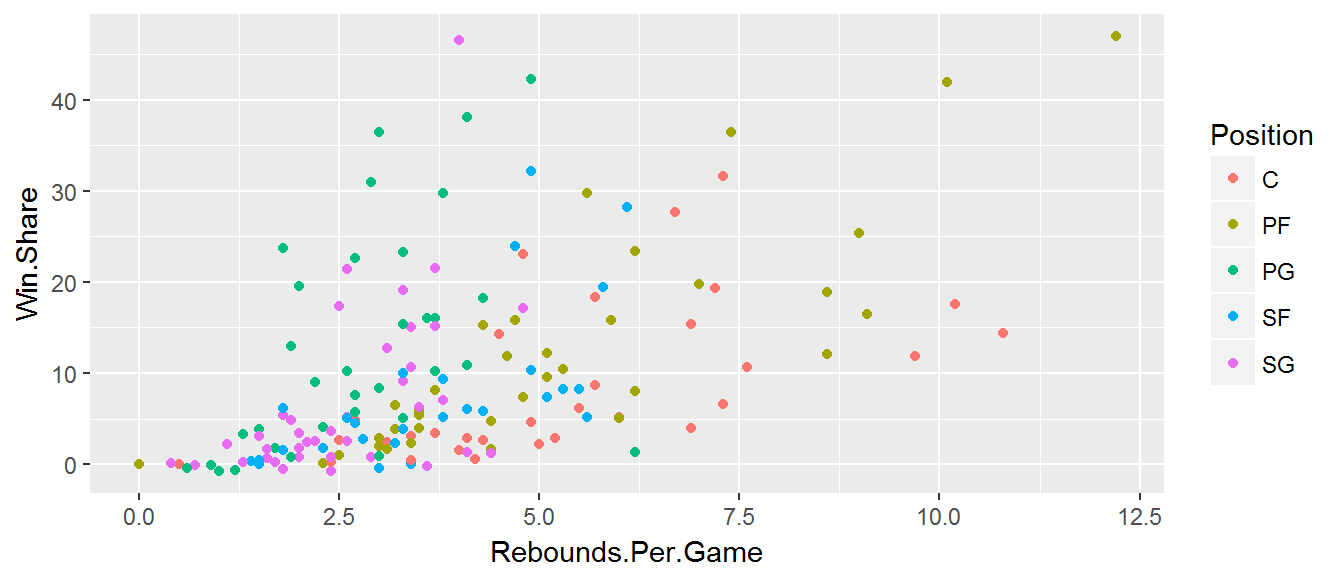1. Edit the plot to add a centered titled and labeled axes without the periods.

2. Change the shape of each point with respect to groups (Lookup documentation if needed).

### 1.

# One of many that will produce the same plot
ggplot(
aes(x = Rebounds.Per.Game, y = Win.Share, colour = Position), data = nba) +
geom_point()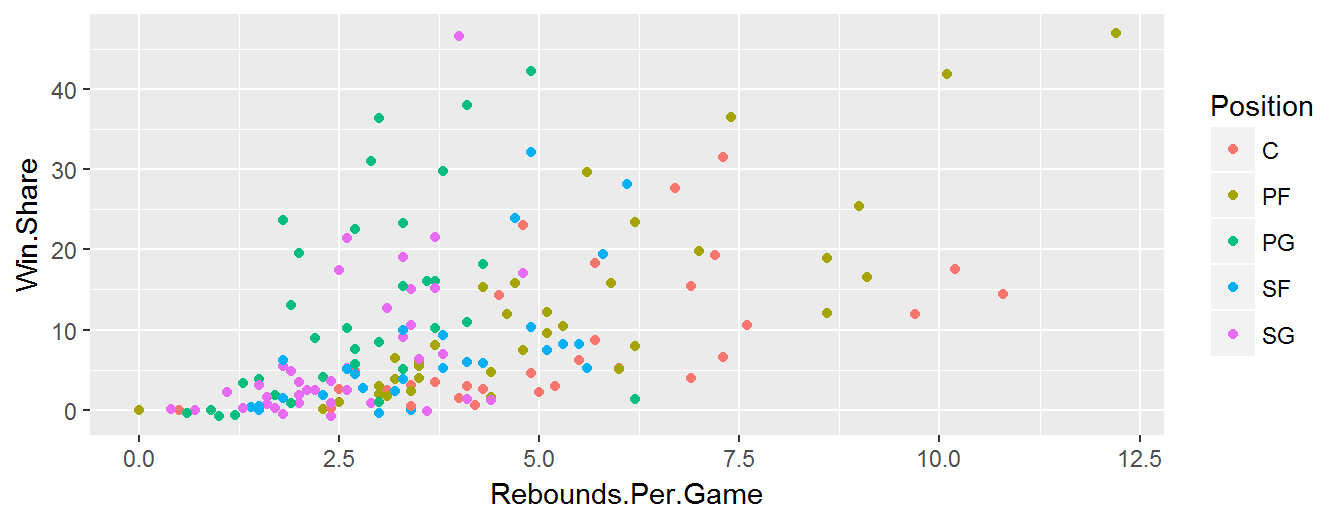### 2.

ggplot(
aes(x = Rebounds.Per.Game, y = Win.Share, colour = Position), data = nba) +
geom_point()+
ggtitle("Win Shares vs. Rebounds Per Game") +
labs(x = " Rebounds Per Game", y = "Win Shares") +
theme(plot.title = element_text(hjust = .5))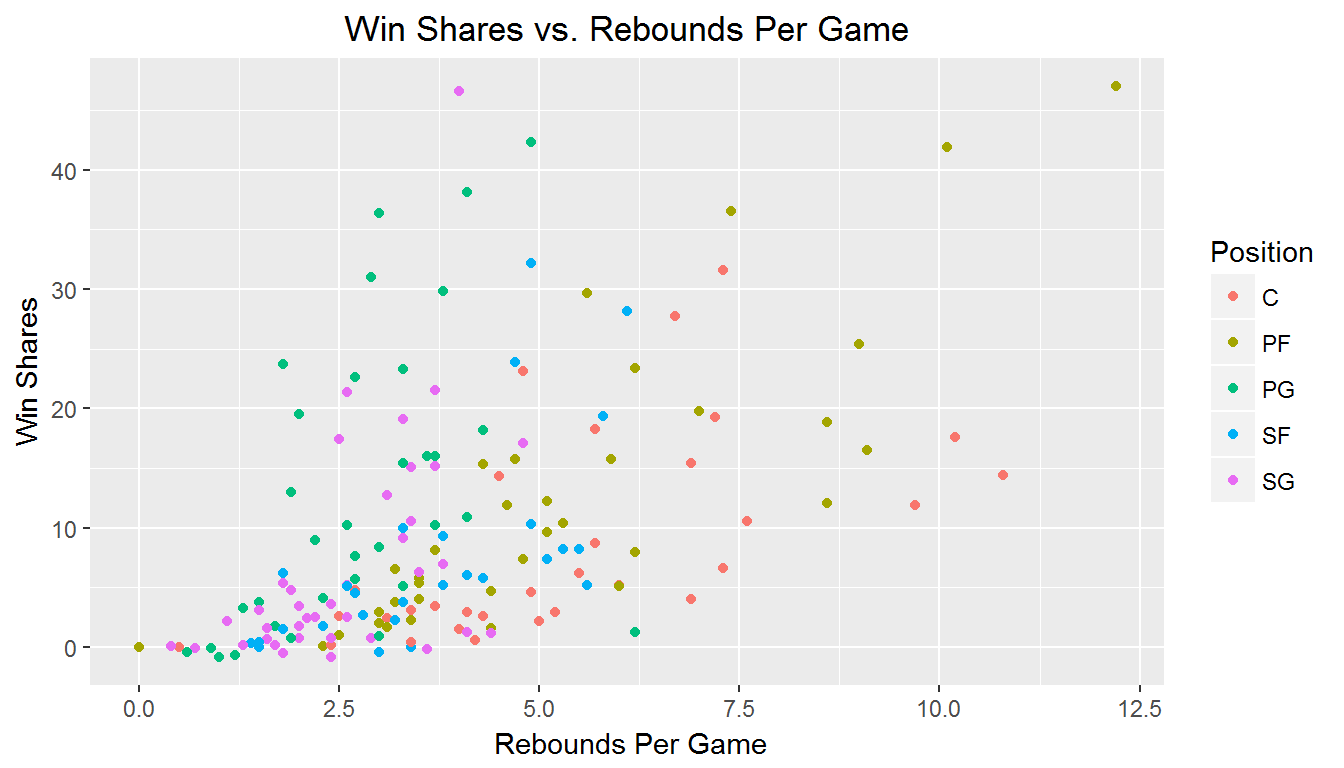### 3.

ggplot(
aes(x = Rebounds.Per.Game, y = Win.Share, colour = Position), data = nba) +
geom_point(aes(shape = Position)) +
ggtitle("Win Shares vs. Rebounds Per Game") +
labs(x = " Rebounds Per Game", y = "Win Shares") +
theme(plot.title = element_text(hjust = .5))